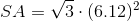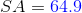## Example Questions

### Example Question #74 : Solid Geometry

If the edge length of a tetrahedron is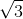, what is the surface area of the tetrahedron?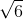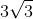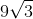Explanation:

Write the formula for finding the surface area of a tetrahedron.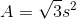Substitute the edge and solve.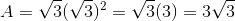### Example Question #15 : How To Find The Surface Area Of A Tetrahedron

Each of the faces of a regular tetrahedron has a base of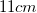and a height of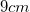. What is the surface area of this tetrahedron?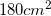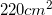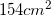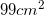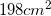Explanation:

The surface area is the area of all of the faces of the tetrahedron. To begin, we must find the area of one of the faces. Because a tetrahedron is made up of triangles, we simply plug the given values for base and height into the formula for the area of a triangle: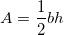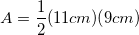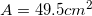Therefore, the area of one of the faces of the tetrahedron is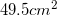. However, because a tetrahedron has 4 faces, in order to find the surface area, we must multiply this number by 4: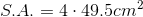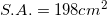Therefore, the surface area of the tetrahedron is.

### Example Question #16 : How To Find The Surface Area Of A Tetrahedron

What is the surface area of a regular tetrahedron with a slant height of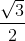?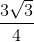Cannot be determined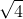Explanation:

If this is a regular tetrahedron, then all four triangles are equilateral triangles.

If the slant height is, then that equates to the height of any of the triangles being.

In order to solve for the surface area, we can use the formula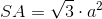wherein this case is the measure of the edge.

The problem has not given the edge; however, it has provided information that will allow us to solve for the edge and therefore the surface area.

Picture an equilateral triangle with a height.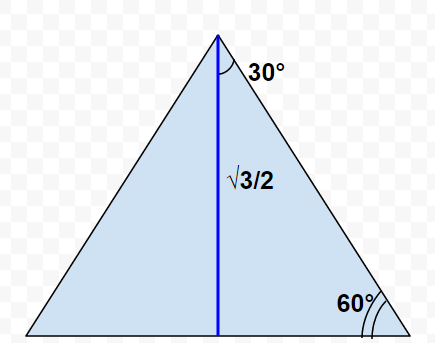Drawing in the height will divide the equilateral triangle into two 30/60/90 right triangles. Because this is an equilateral triangle, we can deduce that finding the measure of the hypotenuse will suffice to solve for the edge length ().

In order to solve for the hypotenuse of one of the right triangles, either trig functions or the rules of the special 30/60/90 triangle can be used.

Using trig functions, one option is using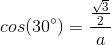.

Rearranging the equation to solve for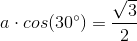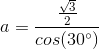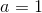Now thathas been solved for, it can be substituted into the surface area equation.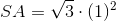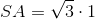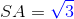### Example Question #17 : How To Find The Surface Area Of A Tetrahedron

What is the surface area of a regular tetrahedron when its volume is 27?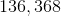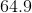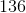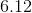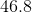Explanation:

The problem is essentially asking us to go from a three-dimensional measurement to a two-dimensional one. In order to approach the problem, it's helpful to see how volume and surface area are related.

This can be done by comparing the formulas for surface area and volume: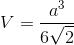We can see that both calculation revolve around the edge length.

That means, if we can solve for(edge length) using volume, we can solve for the surface area.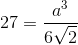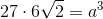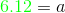Now that we know, we can substitute this value in for the surface area formula: